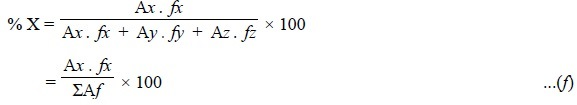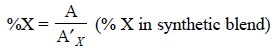Home | | Pharmaceutical Drug Analysis | Working Techniques for Quantitative Analysis - Gas Liquid Chromatography (GLC)

# Working Techniques for Quantitative Analysis - Gas Liquid Chromatography (GLC)

In actual practice, the following three working techniques are not only widely popular but also provide optimum accuracy and precision for the quantitative analysis of pharmaceutical substances, namely : (i) Area Normalization, (ii) Internal Standard Method, and (iii) Comparison Method.

WORKING TECHNIQUES FOR QUANTITATIVE ANALYSIS

In actual practice, the following three working techniques are not only widely popular but also provide optimum accuracy and precision for the quantitative analysis of pharmaceutical substances, namely :

(i) Area Normalization,

(ii) Internal Standard Method, and

(iii) Comparison Method.

There three techniques will be discussed briefly in the sections that follow :

## 1. AREA NORMALIZATION

Assuming that the chromatogram is as represented in Figure 29.6, the formula employed is :where, A = Peak area, and

f = Response factor.Generally, different components possess different response factors, application of which not only com-pensates for different detector response for different components but also take into consideration the other factors inherent with the procedure. However, these factors may be calculated by preparing a synthetic mixture absolutely identical to what is expected in the sample, and subsequently carrying out the gas-chromatography of this mixture exactly under idential experimental parameters as described in the method of analysis. Thus, we have :where, W = Weight or conc. of component in the mixture, and

r = A reference component present in the mixture which is assumed to have response factor of unity.

In certain instances, like petroleum fractions, where it may be possible to assume that most of the components possess almost equal response factors, the area normalization formula in Eq. (f) may be further simplified to :Salient features of Area Normalization Method are as follows :

(i) Very suitable for routine type of samples where the variations in composition are only marginal i.e., in such cases where the response factors need to be checked periodically only when necessary, and

(ii) An obligatory condition of this method being that all the components of the sample should elute and be recorded.

## 2. INTERNAL STANDARD METHOD

In this particular method it is necessary to select a reference compound (known as-internal standard)

that should meet the following requirements rigidly :

(i) It is not a component of the sample but as far as possible, is chemically identical,

(ii) It is resolved from various components of the sample, and

(iii) It elutes near the components of interest.

The internal standard (IS) is usually added to the sample in such a concentration that matches favour-ably with that of components to be evaluated. Now, the respective chromatogram is obtained by the GC-method. The percentage of the sample is obtained by the following expression :.......................(i)

where,         W = Weight of the sample,

w = Weight of the internal standard,

AIS = Peak area of internal standard, and

fIS = Response factor of the internal standard.

Graphical Approach : Many a times a ‘graphical approach’ as illustrated in Figure 29.7 is also applied for the quantitative determination of components in the sample.First and foremost, the calibration curves are plotted for each component by GC-method using synthetic blends (containing varying concentrations of the component and fixed known concentration of IS) and also plotting A/AIS Vs %-concentration. Then running separately the sample (plus IS) in a similar manner and determining A/AIS value, %-concentration of the component may be observed from the calibration curve.

Salient features of Internal Standard Method-are as follows :

(i) It gives very accurate and precise results,

(ii) It completely eliminates possibility of error caused due to loss of some part of the sample (other than the determined components) during the initial preparation stage,

(iii) It eliminates error due to incomplete elution of all the sample components, and

(iv) It eliminates error caused due to inaccurate measurement of sample size before injection.

## 3. COMPARISON METHOD

The ‘comparison method’ makes use of a purely synthetic blend containing the component to be determined in the same order of concentration as expected in the sample. In fact, the very purpose of this synthetic-blends is only to simulate a typical sample. Now, exactly equal (or known) amounts of both, the ‘synthetic blend’ and the ‘sample’are separately injected and chromatograms obtained. Thus, by actually comparing the areas of the desired component in both the chromatograms, the ‘unknown concentration’ may be determined by the following expression :where, AX = Peak area of component X in the chromatogram of ‘synthetic blend’.

However, this method is less accurate in comparison to the first two methods described earlier for quantitative analysis. It should be used judiciously if only a few components present in small concentration (i.e., < 5%) in the sample are required to be estimated e.g., in trace-analysis.

Precautions : Following are certain precautions that must be observed in the quantitative analysis, namely :

(i) Detector response should always be linear in the concentration range covered in the analysis,

(ii) Distortion of the peak caused due to detector and recorder performance must be as negligible as possible,

(iii) Both sample decomposition and adsorption in any portion of the GC-assembly must be avoided, and

(iv) Adequate and precise sampling technique must be followed to permit injection of representative sample only. Obviously, this part of analyis is as vital and critical as the gas chromatorgraphic part of analysis.

Study Material, Lecturing Notes, Assignment, Reference, Wiki description explanation, brief detail
Pharmaceutical Drug Analysis: Gas Liquid Chromatography (GLC) : Working Techniques for Quantitative Analysis - Gas Liquid Chromatography (GLC) |